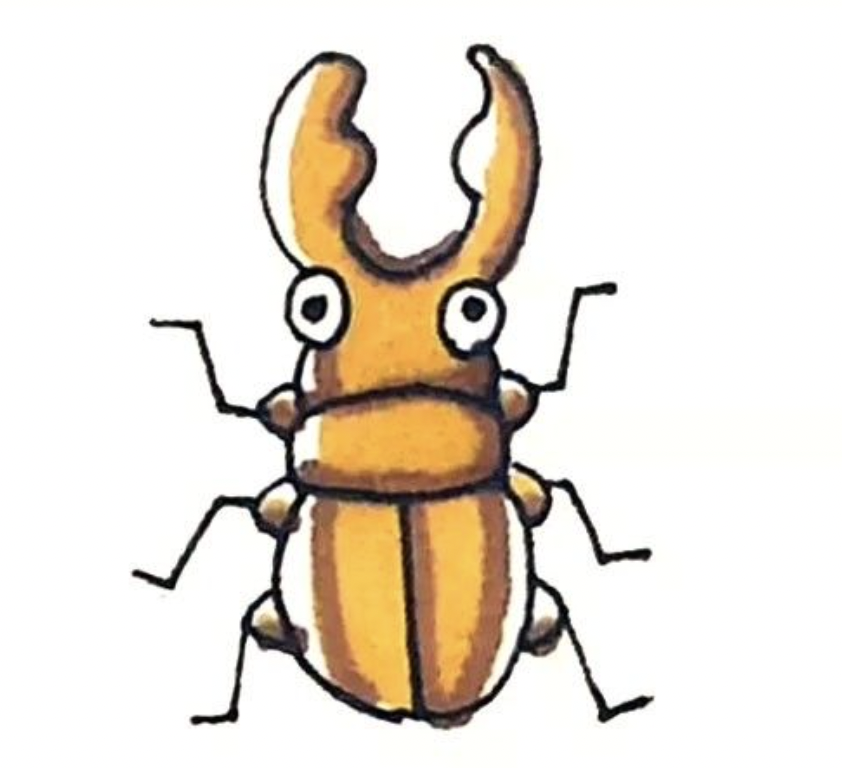V1

2023/03/23阅读：56主题：嫩青

# 用R画个区域图(LocusZoomlikePlot)

## 位点区域图(Regional plot)

A regional association plot is essentially a zoomed-in Manhattan plot, allowing the researcher to look at associations in a small, pre-defined area of the genome.

### 二、 LD 与 GWAS Summary

``plink --bfile ../LTL001/allchr \      --r2 \      --ld-snp-list sig.SNP_ID.txt \ # SNPID list      --ld-window-kb 1000 \ # 滑动窗口大小      --ld-window 99999 \ # 窗口内位点数量最大值      --ld-window-r2 0 \ # 设定最终纳入 LD 结果的位点的 R2 限制      --out 5e-06 # 结果生成 5e-06.ld 文件``

### 三、 R 绘制区域图

R 绘制区域图示例

``library(data.table)library(tidyverse)library(ggsci)assoc <- fread("../GWAS/LTL001_sep/assocall_control")ld <- fread("control5e-06.ld")assoc <- assoc[, c(1, 2, 3, 14)]colnames(assoc) <- c("Chr", "Pos", "SNPID", "P")ld <- ld[which(ld\$SNP_B %in% assoc\$SNPID), ]dat.sig <- assoc[which(assoc\$SNPID %in% ld\$SNP_A), ]dat.sig\$R2.group <- as.factor("5")ld\$sigID <- as.factor(ld\$SNP_A)for (i in 1:length(levels(ld\$sigID))) {    dat <- subset(ld[, c(4, 5, 6, 7, 3)], ld\$sigID == levels(ld\$sigID)[i])    colnames(dat) <- c("Chr", "Pos", "SNPID", "R2", "sigID")    datP <- assoc[which(assoc\$SNPID %in% dat\$SNPID), c(3, 4)]    dat <- merge(dat, datP, by = "SNPID")    dat\$R2.group <- as.factor(ifelse(dat\$R2 < 0.2, "1", ifelse(dat\$R2 < 0.4, "2", ifelse(dat\$R2 < 0.6, "3", ifelse(dat\$R2 < 0.8, "4", "5")))))    dir <- c()    dir <- paste("5e-06LZplots/", dat\$SNPID, "_LZ_plot", ".pdf", sep = "")    ggplot(dat, aes(x = Pos, y = -log(x = P, base = 10), fill = R2.group)) +        geom_point(shape = 21, color = "black", size = 4.5, stroke = 0.75, alpha = 0.8) +        geom_point(data = dat.sig[which(dat.sig\$SNPID %in% dat\$sigID), ], color = "black", size = 2, alpha = 1, stroke = 0.75, shape = 4) +        theme_classic() +        geom_hline(yintercept = 5.30103, color = "#8b0000", lty = 2) +        geom_hline(yintercept = 6.30103, color = "#8b0000", lty = 2) +        scale_fill_manual(            values = c("1" = "#00007B", "2" = "#97CCF6", "3" = "#75FB4C", "4" = "#F2A93B", "5" = "#EA3323")        )    ggsave(file = dir)}``

### 参考资料



Regional Plot: https://rdrr.io/cran/QCGWAS/man/plot_regional.html#:~:text=A%20regional%20association%20plot%20is,defined%20area%20of%20the%20genome.



LocusZoom: http://locuszoom.org/V1